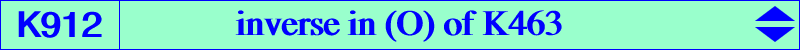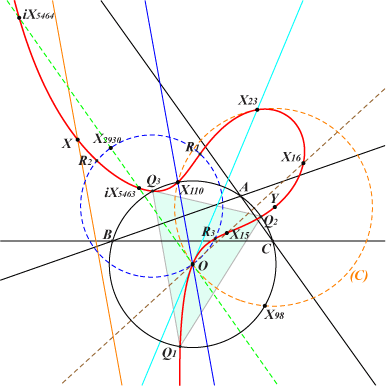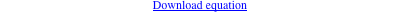too complicated to be written here. Click on the link to download a text file.X(3), X(15), X(16), X(23), X(110), X(5663) inverses in (O) of X(5463), X(5464) : these are X(13858), X(13859) now in ETC (2017-07-13) vertices of the Thomson triangle X(34317), X(34318) : Thomson isogonal conjugates of X(13), X(14) Y on the line X(23)X(5663) and on the perpendicular bisector of X(3)X(110)K912 is the inverse in the circumcircle (O) of K463. It is a focal cubic with singular focus X(23), the inverse of the centroid X(2). The orthic line is X(3)X(110) with infinite point X(5663), hence K912 is the locus of contacts of tangents drawn through X(23) to the circles passsing through X(3) and X(110). In particular, the polar conic (C) of X(23) is the circle passing through X(3), X(23), X(98), X(110), etc, with center X(14270). (C) is the inversive image in (O) of the line passing through X(2), X(98). It is also the polar conic of X(182) in the cubic K009. The real asymptote is the line X(323)X(3663), the image of the orthic line X(3)X(110) under the homothety h(X23, 2). K912 is invariant under the usual conjugation f on a focal cubic which maps a point M to the center of its polar conic with respect to its stelloidal prehessian. Here, if N is the third point of K912 on the line M-X(23) then f(M) is the third point of K912 on the parallel at N to the line X(3)X(110). f swaps (O) and the circle with diameter X(3)X(2930) which must contain the homologues R1, R2, R3 of the vertices Q1, Q2, Q3 of the Thomson triangle. For any M on K912, the segments X(15)X(16) and X(13858)X(13859) are seen under equal or supplementary angles. K1138 is the Thomson isogonal transform of K912. K912 is an isogonal nK with respect to infinitely many triangles. Indeed, if X(3) [resp. X(110)] is one vertex, it is enough to choose the two other vertices of the sought triangle as the two points where a line through X(110) [resp. X(3)] meets K912 again. Examples : X(3), X(23), X(110) or X(15), X(16), X(110). Note that the isogonal conjugation with respect to any triangle T maps each point of K912 to the same point of K912. It obviously transforms the line at infinity to the circumcircle of T. The remaining points of K912 on the sidelines of T are collinear on the trilinear polar (with respect to T) of the root of the cubic. K912 is related to stelloidal pentagonal circum-quartics. See Q125 and specially Q132.